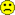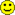#### You may also like### Tweedle Dum and Tweedle Dee

Two brothers were left some money, amounting to an exact number of pounds, to divide between them. DEE undertook the division. "But your heap is larger than mine!" cried DUM...### Sum Equals Product

The sum of the numbers 4 and 1 [1/3] is the same as the product of 4 and 1 [1/3]; that is to say 4 + 1 [1/3] = 4 ï¿½ 1 [1/3]. What other numbers have the sum equal to the product and can this be so for any whole numbers?### Special Sums and Products

Find some examples of pairs of numbers such that their sum is a factor of their product. eg. 4 + 12 = 16 and 4 × 12 = 48 and 16 is a factor of 48.

# Keep it Simple

### Why do this problem?

This is the first problem in a set of three linked activities. Egyptian Fractions and The Greedy Algorithm follow on.

It's often difficult to find interesting contexts to consolidate addition and subtraction of fractions. This problem offers that, whilst also requiring students to develop and analyse different strategies and explain their findings.

### Possible approach

Pose the initial part of the problem as it is set and ask the students to suggest what Charlie's rule might be. Allow some time for them to work out which sums are correct and ask them to modify Charlie's rule so that it always generates correct solutions. Working in pairs, invite students to generate some more examples that confirm their new rule. Collect some of these on the board for a general discusion. (With some classes this could lead to an algebraic explanation/proof.)

Alison's question offers an opportunity to involve the whole class in a collaborative activity. Talk through what Alison might have been thinking as she generated different pairs which worked. This might be an opportunity to talk to the class about the value of working systematically. How can Alison be sure that she has found all the possible pairs?
In pairs, ask students to choose their own unit fraction and find all the correct pairs.
Collect all results on the board and encourage students to share their strategies for finding all possible combinations.

### Key questions

Can a unit fraction always be written as the sum of two different unit fractions?
Which unit fractions can only be written in one way?
What is the strategy for finding all the combinations of two unit fractions that add up to a third unit fraction?

### Possible support

Some students may find it easier to contribute to the class discussion by working systematically to generate lots of unit sum calculations and highlighting any that result in a unit fraction as an answer.

For example

 $\frac{1}{6} + \frac{1}{7} = \frac{13}{42}$$\frac{1}{6} + \frac{1}{8} = \frac{7}{24}$... $\frac{1}{6} + \frac{1}{12} = \frac{1}{4}$### Possible extension

Ask students to produce an algebraic or visual proof of Charlie's revised rule.

Can they predict how many different pairs of unit fractions will add up to any given unit fraction?

You may wish to move students on to Egyptian Fractions.